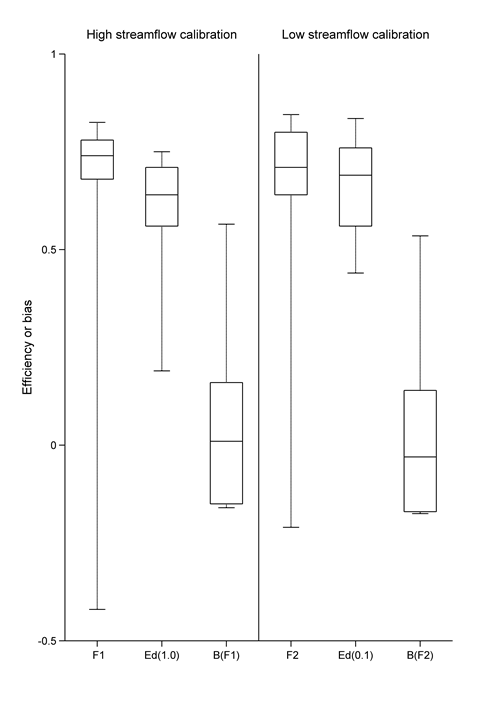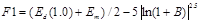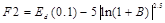# 2.6.1.4.2 Model calibration results

Page 17 of 41

Figure 8 and Table 4 summarise the regional model calibration results for the nine streamflow gauging stations. The bottom, middle and top of each box in Figure 8 represent the 25th, 50th and 75th percentiles, and the bottom and top whiskers represent the 10th and 90th percentiles. For both high-streamflow and low-streamflow calibrations, three metrics (F value, daily efficiency and model bias) are shown and their details are introduced in the Figure 8 notes. Details of each streamflow gauge metric are given in Table 4.

The high-streamflow calibration (F1) yields an overall reasonable Nash–Sutcliffe efficiency of daily streamflow (Ed(1.0)) and low model bias, indicated by a median Ed(1.0) of 0.64 and a median bias of 0.01. This is despite a poor calibration performance at streamflow gauge 203030 (0.08). The values of F1 are also encouraging (with a median of 0.74), with the exception of streamflow gauge 204043, and to a lesser extent streamflow gauge 203030. The reason for the poorer model performance at these two streamflow gauges is not known.

The low-streamflow calibration (F2) is evaluated against the daily streamflow data transformed with a power of 0.1, or a Box‑Cox lambda value of 0.1 (Box and Cox, 1964), which can make sure the model evaluation is putting more weight on low streamflow than higher streamflow. The low-streamflow calibration yields overall good efficiency with the Box‑Cox lambda value of 0.1 (Ed(0.1)), indicated by a median Ed(0.1) of 0.69. Note that streamflow gauge 203030 has a higher Ed(1.0) of 0.59 for the low-streamflow calibration indicating a better calibration using this objective function. The values of F2 are also encouraging (with a median of 0.71), with the exception of streamflow gauge 204043, and to a lesser extent streamflow gauge 203030.Figure 8 Summary of two AWRA-L model calibrations for the Richmond river basin (period is 1 January 1983 to 31 December 2013)

AWRA-L = Australian Water Resources Assessment landscape model

Left: high-streamflow calibration; right: low-streamflow calibration. In each boxplot, the bottom, middle and top of the box are the 25th, 50th and 75th percentiles, and the bottom and top whiskers are the 10th and 90th percentiles. F1 is the F value for high-streamflow calibration; F2 is the F value for the low-streamflow calibration; Ed(0.1) is the daily efficiency with a Box‑Cox lambda value of 1.0; Ed(0.1) is the daily efficiency with a Box‑Cox lambda value of 0.1; B is model bias.

Data: Bureau of Meteorology (Dataset 1, Dataset 2) and Bioregional Assessment Programme (Dataset 4)

Table 4 Summary of model calibration for nine calibration streamflow gauging stations in the Clarence-Moreton bioregion (1 January 1983 to 31 December 2013)

Streamflow gauge ID

Modelled mean annual streamflow (mm/y)

F1a

Ed(1.0)a

Bias (F1)a

F2b

Ed(0.1)b

Bias (F2)b

201005

691

0.68

0.64

–0.16

0.71

0.76

–0.17

203002

905

0.74

0.71

–0.15

0.77

0.84

–0.17

203005

303

0.85

0.78

0.01

0.83

0.76

–0.03

203010

455

0.78

0.64

–0.04

0.80

0.69

–0.06

203014

822

0.80

0.72

0.07

0.86

0.83

0.03

203030

201

0.12

0.08

0.37

0.44

0.59

0.33

203041

205

0.68

0.56

0.16

0.66

0.49

0.14

204043

238

–0.96

0.30

0.76

–0.86

0.39

0.74

204067

218

0.74

0.71

–0.16

0.64

0.56

–0.18

Median

303

0.74

0.64

0.01

0.71

0.69

–0.03

ais the F value for high-streamflow calibration, where Ed(1.0) is the daily efficiency with a Box-Cox lambda value of 1.0, Em is the monthly efficiency and B is the bias.

bis the F value for low-streamflow calibration, where Ed(0.1) is the daily Nash–Sutcliffe efficiency (NSE) with a Box-Cox lambda value of 0.1.

Data: Bureau of Meteorology (Dataset 1, Dataset 2) and Bioregional Assessment Programme (Dataset 4)

The calibration results show that the model can be calibrated against both high-flow and low-flow objective functions with reasonable results, as evidenced by both the median F values and median model efficiencies of around 0.6 to 0.7, and with median bias at low levels. An F value of unity would indicate a perfect fit, with F values approaching minus infinity reflecting poorer and poorer fits.

Last updated:
18 October 2018